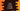# 4 different ways to convert a string to double in Java## 4 different ways to convert a string to Double in Java :

Converting a string to double in Java is easier than you think. We have a lot of different approaches to do this conversion. In this tutorial, we will learn four different ways to convert a string to double. We will include different examples for each. Let’s have a look :

### Using Double.valueOf(String s) :

static method valueOf can be used to convert a string to Double. It takes one string as an argument and returns a Double object that holds the double value represented by the argument string.

If the argument string is null, it throws one NullPointerException and if the argument is invalid, it throws NumberFormatException. The declaration of the valueOf method is as below :

``````public static Double valueOf(String s)
throws NumberFormatException
``````

Let me show you with a simple example :

``````class Example {
public static void main(String args[]) {
String s = "435.23";
Double d = Double.valueOf(s);

System.out.println("Double value is " + d);
}
}
``````

It will print the below output :

``````Double value is 435.23
``````

So, as you can see the string is converted to a Double by the valueOf method.

### Using Double.parseDouble(String s) :

Similar to the above example, parseDouble is also a different static method to convert a string to a double. The only difference between these two methods is that valueOf returns one Double instance but parseDouble returns one primitive double value. The declaration of the parseDouble is as below :

``````public static double parseDouble(String s)
throws NumberFormatException
``````

If the string parameter is null, it will throw one NullPointerException and if the string is not parceable, it will throw one NumberFormatException.

Example :

``````class Example {
public static void main(String args[]) {
String s = "435.230";
double d = Double.parseDouble(s);

System.out.println("Double value for 435.230 is " + d);
System.out.println("Double value for 43.530d is " + Double.parseDouble("43.530d"));
System.out.println("Double value for +21.45 is " + Double.parseDouble("+21.45"));
System.out.println("Double value for -34.54 is " + Double.parseDouble("-34.54"));
}
}
``````

It will print the below output :

``````Double value for 435.230 is 435.23
Double value for 43.530d is 43.53
Double value for +21.45 is 21.45
Double value for -34.54 is -34.54
``````

As you can see from this example that we can also extract double values for positive and negative numbers as well.

### Using new Double(String s) :

This is another way to convert a string to double: using the constructor of Double. Note that this method is deprecated in Java 9. So, it not a recommended way to do the conversion. It returns one Double value of the string parameter passed to the conversion. Example :

``````class Example {
public static void main(String args[]) {
String s = "435.230";
Double d = new Double(s);

System.out.println("Double value for 435.230 is " + d);
}
}
``````

It will print the below output :

``````Double value for 435.230 is 435.23
``````

As you can see on the above image that the compiler is showing one warning message of deprecation. So, even if you are not using Java 9, first and the second methods are more preferable.

### Using DecimalFormat :

DecimalFormat class is useful to convert a number to a string or a string to a number. Example :

``````import java.text.DecimalFormat;
import java.text.ParseException;

class Example {
public static void main(String args[]) {
String s = "435.230";
try {
DecimalFormat decimalFormat = new DecimalFormat("#");
double d = decimalFormat.parse(s).doubleValue();

System.out.println("Double value for 435.230 is " + d);
} catch (ParseException e) {
e.printStackTrace();
}
}
}
``````

The output is as below :

``Double value for 435.230 is 435.23``

The parse() method returns a Number. We need to call doubleValue() method on it to convert it to a double.

### Conclusion :

We have seen four different ways to convert a string to double in Java. First and the second methods are mostly used. Try to run the examples with different inputs and drop one comment below if you have any queries.